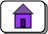Created: 08/07/03

Basic MIPS Instructions

These are the assembly language statements covered in these notes that each directly correspond to one machine language instruction. There are additional basic assembly language statements that are not covered in these notes.

When pseudoinstructions are enabled, many of these instructions also correspond to pseudoinstructions that have greater flexibility in the arguments that they allow.

In the following, d, s, and t are general purpose registers. Register d receives the result of operation between the contents of registers s and t .

InstructionOperandsDescription
d <-- s+t ; with overflow trap
d <-- s+t ; without overflow trap
d <-- s+const ; with overflow trap
const is 16-bit two's comp
d <-- s+const ; without overflow trap
const is 16-bit two's comp
and d,s,t
d <-- bitwise AND of s with t
andi d,s,const
d <-- bitwise AND of s with const
branch if s == t
A branch delay slot follows the instruction.
Branch if the two's comp. integer
in register s is >= 0
A branch delay slot follows the instruction.
Branch if the two's comp. integer
in register s is < 0
A branch delay slot follows the instruction.
branch if s != t
A branch delay slot follows the instruction.
div s,t
lo <-- s div t ; hi <-- s mod t
two's comp. operands
divu s,t
lo <-- s div t ; hi <-- s mod t
unsigned operands
j target
after a delay of one machine cycle,
lb d,off(b)
d <-- Sign-extended byte from
off is 16-bit two's complement
lbu d,off(b)
d <-- Zero-extended byte
off is 16-bit two's complement
lh d,off(b)
t <-- Sign-extended halfword
off is 16-bit two's complement
lhu d,off(b)
t <-- Zero-extended halfword
off is 16-bit two's complement
lui d,const
upper two bytes of \$t <-- two byte const
lower two bytes of \$t <-- 0x0000
lw d,off(b)
d <-- Word from memory address b+off
off is 16-bit two's complement.
mfhi d
d <-- hi ;  Move From Hi
mflo d
d <-- lo ;  Move From Lo
mult s,t
hi / lo < -- s * t ;  two's comp operands
multu s,t
hi / lo < -- s * t ;  unsigned operands
nor d,s,\$0
d <-- bitwise NOT of s
nor d,s,t
d <-- bitwise NOR of s with t
or d,s,\$0
d <-- s
or d,s,t
d <--bitwise OR of s with t
ori d,\$0,const
d <-- zero-extended const
ori d,s,const
d <-- s OR zero-extended const

sb d,off(b)
byte at off+b <-- low-order byte
from register \$d.
off is 16-bit two's complement
sh d,off(b)
two bytes at off+b <-- two low-order bytes
from register \$d.
off is 16-bit two's complement
sll \$0,\$0,0
no operation
sll d,s,shft
d <-- logical left shift of s by shft positions
where  0 <= shft < 32
slt d,s,t
if s < t
d <-- 1
else
d <-- 0

two's comp. operands
slti d,s,imm
if s < imm
d <-- 1
else
d <-- 0

two's comp. operands
sltiu d,s,imm
if s < imm
d <-- 1
else
d <-- 0

unsigned operands
sltu d,s,t
if s < t
d <-- 1
else
d <-- 0

unsigned operands
sra d,s,shft
d <-- arithmetic right shift of s by shft positions
where  0 <= shft < 32
srl d,s,shft
d <-- logical right shift of s by shft positions
where  0 <= shft < 32
sub d,s,t
d <-- s - t; with overflow trap
subu d,s,t
d <-- s - t; no overflow trap
sw d,off(b)
Word at memory address (b+off) <-- \$t
b is a register. off is 16-bit twos complement.
xor d,s,t
d <-- bitwise exclusive or of s with t
xori d,s,const
d <-- bitwise exclusive or of s with constReturn to Home